# Carbon fibre tube -- is round or oval stronger?

Karl christophersen
Hi all, my question is if i have a length of carbon fibre tube, one is round the other is oval and orientated along its longest side is it more stiff than the round one? Reason for asking is i am trying to make a telescopic carbon fibre pole that is more rigid than the round ones on the market. The object on the top of the pole will keep the orientation of the pole at the correct position.

#### Attachments

•Oval-Carbon-Fiber-and-Fiberglass-Tube.jpg
36.2 KB · Views: 684

DaveC426913
Gold Member
I am a layperson, not an engineer or mathematician.

For a given circumference, compared to a circle, the elliptical cross section will be stronger through its semi-major axis and weaker through its semi-minor axis.

If the primary bending load is in a predictable direction, you can align the semi-major axis that way. (i.e. if your pole can be kept oriented so its semi-majr axis is vertical, to oppose gravity).

If the bending load is not predictable, or is not evenly distributed (i.e. if the pole can be rotated around its aixs), you'll have a problem - a column is only going to be as strong as its weakest cross-section, so it will be more prone to failure than a comparable circular cross-section.

•Mech_Engineer, Asymptotic and Karl christophersen
Karl christophersen
I am a layperson, not an engineer or mathematician.

For a given circumference, compared to a circle, the elliptical cross section will be stronger through its semi-major axis and weaker through its semi-minor axis.

If the primary bending load is in a predictable direction, you can align the semi-major axis that way. (i.e. if your pole can be kept oriented so its semi-majr axis is vertical, to oppose gravity).

If the bending load is not predictable, or is not evenly distributed (i.e. if the pole can be rotated around its aixs), you'll have a problem - a column is only going to be as strong as its weakest cross-section, so it will be more prone to failure than a comparable circular cross-section.

DaveC426913
Gold Member
It occurs to me that some experimentation with lengthwise fluting/corrugation might help with stiffness.
I think you'd wouldn't need them all the way around, just at the semi-minor axis, to add stiffness there.

But I've never seen this done, and I'm just spitballing.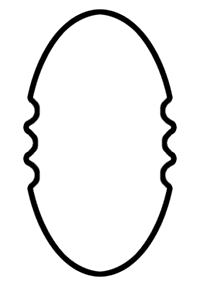Last edited:
DaveC426913
Gold Member
A question occurs to me though:

What is the advantage of yours intended to be over a circular cross-section?

A large diameter tube is stiffer than a small diameter tube, so if strength were the only consideration, there'd be no need to change it's shape. You'd just use a wider diameter tube (it wouldn't need to have thicker walls). This is not what you want, of course.

Clearly, the goal then must be to achieve similar strength but with less weight.

And that raises the question: just how much weight do you think you could save your potential customer?

An elliptical cross-section with a 2:1 ratio would use only 71% of the material as that of a round tube (at a cost to strength of [ ?? ]).
Let's assume an ideal case that you find a design where the strength is not compromised i.e. it equivelant to the same diameter circular tube.

Would your 142g pole really outcompete their 200g pole?

(I guess, if weight were a premium, such as ultra-light craft, sure. But I get the impression that's not your application.)

Last edited:
Nidum
Gold Member
@Karl christophersen

There is no simple general answer to this question . Answer depends on several different factors - any of which may or may not apply here .

DaveC426913
Gold Member
There is no simple general answer to this question . Answer depends on several different factors - any of which may or may not apply here .
I dunno. I think we can infer a general picture.

Think of a selfie-stick for a camera. It is meant to be rigid against gravity. In the OP's case, for some reason, we can assume the stick stays oriented around its long axis. i.e it does not rotate about its long axis (in aeronautical terms this is called roll).

So, an elliptical cross-section could be as strong vertically (i.e. pitch) as a circular cross-section, yet need not be as strong in the transverse direction (i.e. yaw). Thus, he could save a bit of weight.

An ideal configuration would make it equally as strong as a circular section, but that might not be achievable in practice. Nonetheless, the OP's stick would have a higher strength-to-weight ratio.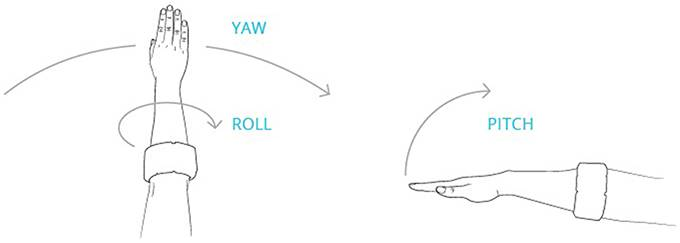Last edited:
Mech_Engineer
Gold Member
The best method for comparing bending strength of different cross-sectional shapes is to use the "section modulus," also sometimes called the "section area moment of inertia" (although this parameter doesn't have anything to do with inertia).

An example list of section moduli properties can be found here: http://www.engineersedge.com/section_properties_menu.shtml. The two sections you're most interested in are the hollow circular tube (Case 19) and the elliptical hollow tube (Case 22). The hollow eccentric tube will have a higher section modulus in one direction, mirroring DaveC's previous post regarding strength along the semi-major and semi-minor axis.

My copy of Roark's Formulas for Stress and Strain (Seventh Edition) has formulas for each case.

For a hollow circular tube: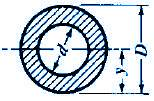$$I_{x} = I_{y} = \frac{π}{4}(R^{4}-R_{i}^{4})$$

Hollow elliptical tube (internal hole is elliptical too, so non-constant wall thickness):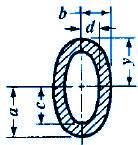$$I_{x} = \frac{π}{4}(ba^{3}-b_{i}a_{i}^{3})$$
$$I_{y} = \frac{π}{4}(ab^{3}-a_{i}b_{i}^{3})$$

I agree with Mech_Engineer's analysis regarding the pole's rigidity.

As an aside, I like DaveC's suggestion about corrugating the pole. The problem would be manufacturing, specifically making sure that the radii are large enough that the CF can fill in the grooves and not be smaller than the minimum bend radius. Also, I think the manufacturing cost of using more complicated shapes with CF will be prohibitive since a "normal" pole is either probably filament wound or even just rolled up prepreg. If cost is no object, because it's carbon fiber you could get away with making more complicated structures, like this: http://www.isotruss.com/. Depends on the ultimate goal.

Karl christophersen
Without giving too much away about potential applications as this picture shows the pole can maintain its position a chosen axis with minimal lateral pressure. Mech engineers technical formula lost me to be honest, if i could only decipher the formula. My goal was to increase rigidity by approximately 25%.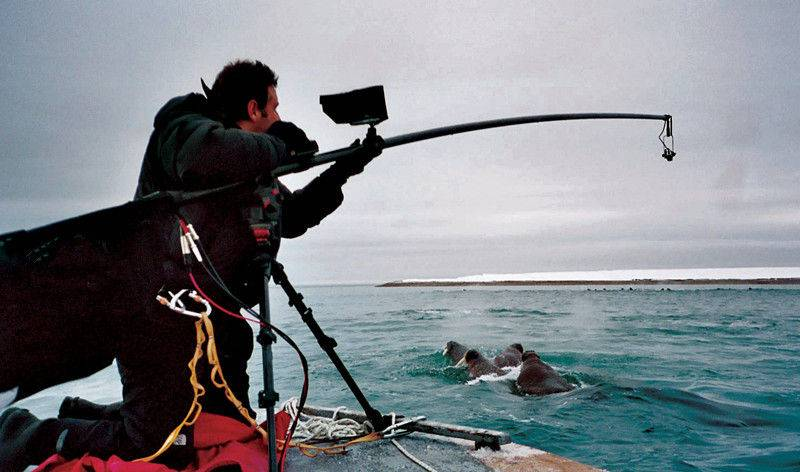Nidum
Gold Member
As I said earlier there are many different issues to consider . The primary one is that changing to an oval section might increase the stiffness in the vertical sense but it would also reduce the lateral stiffness . This means that any lateral forces acting on the rod would cause it to flex more .

For the same weight of material used a properly designed hollow tube can be much stiffer in both directions than a solid rod .

The practical answer to your problem may be in having the inboard sections of the boom of larger diameter hollow tube and outer sections of solid rod .

Easy enough to analyse a variable section boom . If you would like to sketch out something along the lines suggested above or based on your own ideas we can have a look at it for you .

DaveC426913
Gold Member

OK. OK. Hold the phone.

The cross section you posted is an ellipse that is horizontal. That is its weakest orientation with respect to gravity.

BUT -
that's not the point of it, is it?

The horizontal orientation is intended to induce an arch in the rod, which actually provides lateral (roll) stability. (The rod cannot rotate around its long axis as long as it is arched):That is a completely different kettle of fish than mere rigidity against gravity.

Apologies to @Nidum . S/he was correct is saying we don't have nearly enough information.

Karl christophersen
Sorry the ellipse illustration was merely showing the shape i thought would be good, not the orientation. I thought if i could make a pole with the longer side about 20% more than the shorter side i would be able to increase it's strength along its long side whilst minimising loss of rigidity on the shorter side. Here is the use of the pole i had in mind, as you can see the majority of the force is one direction.
These poles get used up to seven stories high and after about 3 stories a lot of pressure is lost through the flex in the pole, there is very minimal sideways pressure.

Nidum
Gold Member
An answer to that problem could be to have secondary support to reduce the flexing .

Can't make specific recommendation without knowing how this system is mounted and controlled at the bottom but conceptually it could be arrangements like this :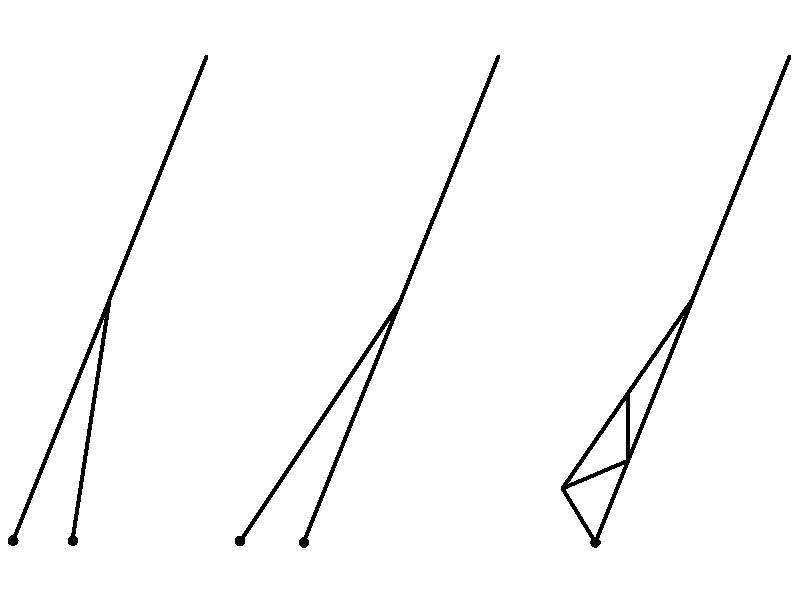Karl christophersen
This should make it clearer

Mech_Engineer
Gold Member
Why not just use a larger overall circular tube of carbon fiber? The added complexity of fabrication and design for an elliptical profile might be hard to justify for relatively small weight savings.

DaveC426913
Gold Member
Why not just use a larger overall circular tube of carbon fiber? The added complexity of fabrication and design for an elliptical profile might be hard to justify for relatively small weight savings.
Agree. This was the gist of my post #5.

Mech_Engineer
Gold Member
So take for example the following thought experiment. Take a pole with the following parameters (for simplicity we'll look at a single diameter rather than a nested collapsible design):
• Length: 30 ft
• Outer Diameter: 1.50 in
• Inner Diameter: 1.38 in (wall thickness of 1/16")
• Material: Layered carbon fiber (approx. density of 0.92 oz/in^3, E = 33x10^6 psi)
This tube has:
• Section modulus of 0.073 in^4
• Weight of about 3.11 oz/ft (5.84 lb for the 30' tube)
• Held horizontally cantilever would bend down about 4.95" due to gravity (not bad for a 30' cantilever pole)

If you take another tube and increase its diameter by 0.5" with the same wall thickness (OD = 2.000", ID = 1.875")
• Section modulus of 0.179 in^4
• Weight of about 4.20 oz/ft (7.87 lb for the 30' tube)
• Held horizontally cantilever would bend down about 2.73" due to gravity
So in this example a 33% increase in diameter results in a 145% increase in stiffness, and a 45% decrease in sag for a 30 foot pole.

Karl christophersen
So take for example the following thought experiment. Take a pole with the following parameters (for simplicity we'll look at a single diameter rather than a nested collapsible design):
• Length: 30 ft
• Outer Diameter: 1.50 in
• Inner Diameter: 1.38 in (wall thickness of 1/16")
• Material: Layered carbon fiber (approx. density of 0.92 oz/in^3, E = 33x10^6 psi)
This tube has:
• Section modulus of 0.073 in^4
• Weight of about 3.11 oz/ft (5.84 lb for the 30' tube)
• Held horizontally cantilever would bend down about 4.95" due to gravity (not bad for a 30' cantilever pole)

If you take another tube and increase its diameter by 0.5" with the same wall thickness (OD = 2.000", ID = 1.875")
• Section modulus of 0.179 in^4
• Weight of about 4.20 oz/ft (7.87 lb for the 30' tube)
• Held horizontally cantilever would bend down about 2.73" due to gravity
So in this example a 33% increase in diameter results in a 145% increase in stiffness, and a 45% decrease in sag for a 30 foot pole.

Interesting, the main issue with this is the increase in pole diameter increases weight and usability.

Karl christophersen
There are designs out in the market that use large diameter poles that are the same size but each time you need to raise or lower your pole as you wash down the building attaching or detaching pole is very cumbersome. Telescopic is the way to go as it collapses easily and is the most productive, the problem is its flex.

Tom.G
In post #13
after about 3 stories a lot of pressure is lost through the flex in the pole
I beg to differ. The loss of force at the far end is caused by simple lever action. The operator is grasping the pole with his hands perhaps three feet apart. Lets assume the lower end of the pole is held steady and three feet above that, the operator is pushing with ten pounds of force. If the pole is six feet long there is only five pounds of force at the far end. At three stories high, the pole may be 36 feet long. The force at the far end of that 36 foot pole is now (3ft./36ft) x 10lbs. = (1/12) x 10lbs = 0.833lbs = 13Ounces.

That's why a crowbar works, it's a simple lever where Force times Distance is a constant. In the window washing scenario the operator is at the short end of the crowbar and the brush is at the long end.Nidum
Gold Member
Yes part of the problem is the lever length .

With shorter extension lengths the operator could compensate for any flexing by applying more load at the bottom of the rod .

As the extension length gets greater applying more load at the bottom of the rod to compensate for flexing becomes progressively more difficult and less effective .

Solution to problem for long extension lengths requires more stiffness in the rod and a more effective way for user to apply load at the bottom .

These problems together can be solved by the use of a lightweight support structure for the rod and by devising a more effective hand hold .

Last edited: